# Glasses

There are 36 pupils in the class. Nine girls wear glasses. Boys with glasses are five less than girls without glasses. Boys without glasses are two times more than girls without glasses. How many boys and how many girls?

c =  19
d =  17

### Step-by-step explanation:

c+d = 36
d = 9 + f
c = a + b
a = f - 5
b = 2 f

c+d = 36
d = 9 + f
c = a + b
a = f - 5
b = 2•f

c+d = 36
d-f = 9
a+b-c = 0
a-f = -5
b-2f = 0

a = 3
b = 16
c = 19
d = 17
f = 8

Our linear equations calculator calculates it.
$d=17=17$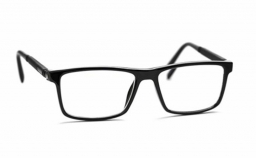Did you find an error or inaccuracy? Feel free to write us. Thank you!Tips to related online calculators
Do you have a system of equations and looking for calculator system of linear equations?

## Related math problems and questions:

• PupilsThere are 32 pupils in the classroom, and girls are two-thirds more than boys. a) How many percents are more girls than boys? Round the result to a whole percentage. b) How many boys are in the class? c) Find the ratio of boys and girls in the class. Writ
• In the classroomThere are 30 boys and a few girls in the class. In the six months, 28 boys and all girls benefited, which was 95% of all pupils. How many pupils are there in the classroom?
• Prospering studentsThere are girls and 30 boys in the class. Boys prospering 28, girls all. How many girls are in the class and how many boys are there, if all the prospering students are 95%?
• ClassroomThere are eighty more girls in the class than boys. Boys are 40 percent and girls are 60 percent. How many are boys and how many girls?
• Classroom 4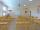In a class of 36 pupils, 2/3 are girls. How many girls and boys are in the class?
• Classroom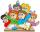In a class are 32 pupils. Boys are 8 less than girls. How many boys and girls are in the classroom?
• Classroom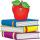In a classroom are 30 students, boys are four times more than girls. How many boys are in the class?
• Boys and glasses2/3 of the students in Sarah’s class are boys. Of the boys, 1/3  of them wear glasses. What fraction of the students wear glasses?
• A class IV.CIn a class 2/5 were boys. 30 were girls. How many more girls than boys are there?
• GirlsThe boys and girls in the class formed groups without the rest of the fives, two girls and three boys. There are six girls missing to create mixed pairs (1 boy and one girl). How many girls are in the classroom?
• ChildrenThe group has 42 children. There are 4 more boys than girls. How many boys and girls are in the group?
• School year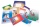At the beginning of the school year, 396 notebooks and 252 textbooks are ready to be distributed in the classroom. All pupils receive the same number of notebooks and the same amount of textbooks. How many pupils are there in the class if you know that th
• Boys and girlsThere are eight boys and nine girls in the class. There were six children on the trip from this class. What is the probability that left a) only boys b) just two boys
• Eight chairsThere are eight chairs by the table and one more child sitting on each one. There are two times more girls than boys. How many girls and how many boys can there be?
• Boys and girls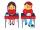In the class are 20 boys and 5-times less girls. How many girls are in the classroom? How many all children are in the class?
• PupilsThere are 350 girls in the school, and the other 30% of the total number of pupils are boys. How many pupils does the school have?
• Boys and girlsThere are 48 children in the sports club, boys are 10 more than girls. How many girls go to the club?